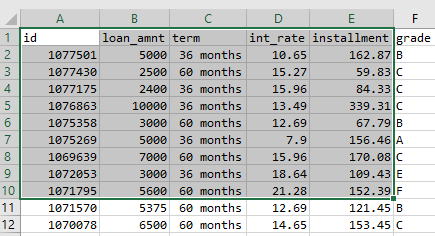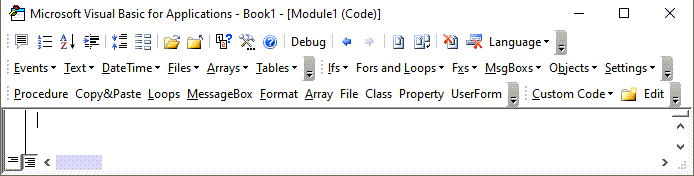# Excel VBA Resize Range

## Resize Range Using VBA

This tutorial will demonstrate how to use the Resize Property of the Range Object to change to return a new range resized from the original Range object.

### Syntax

Syntax for the Resize property is:

``Range("A1").Resize (RowSize, ColumnSize)``

Where Range(“A1”) is your starting range.

RowSize and ColumnSize must be greater than zero. Each input is optional (ex. you can omit RowSize to only change the number of columns, or vice-versa).

### Resize Number Of Rows And Columns

Following example expands the range with one cell A1 to range A1:D10 by increasing row count to 10 and columns count to 5.

``Range("A1").Resize(10, 5).Select``Or, more commonly you’ll assign the resized range to a variable:

``````    ' Resize range to the desired size and assign to a variable
Set newRng = ActiveSheet.Range("A1").Resize(10, 5)``````

### Resize Number Of Rows Only

Following example changes the number of rows only:

``````    ' Change Row Size only, New Range will be \$A\$1:\$A\$10
Set newRng = rng.Resize(10)
``````

### Resize Number Of Columns Only

Following example changes the number of columns only:

``````    ' Change Column Size only, new Range will be \$A\$1:\$E\$1
Set newRng = rng.Resize(, 5)``````

### Resize Table Range To Exclude Header

If you have a table on active sheet with a header row, the code will first select the entire table then move down one row to exclude the header using Range.Offset method. It will then use the Range.Resize property to reduce the size by one row.

``````Sub SelectTableData()
' **IMPORTANT**
' Click on any cell of the table before running the macro

' Move down one row by using Offset and then reduce range size by one row
Set tbl = ActiveCell.CurrentRegion.Offset(1, 0)
Set tbl = tbl.Resize(tbl.Rows.Count - 1, tbl.Columns.Count)

' Data is selected excluding header row
tbl.Select
End Sub
``````

Stop searching for VBA code online. Learn more about AutoMacro - A VBA Code Builder that allows beginners to code procedures from scratch with minimal coding knowledge and with many time-saving features for all users!### Write 2-D Array To Range

Another common usage is to write a 2 dimensional array to a sheet. Since the range to be written should match the size of the array, which is normally not known in advance, the Resize method is used to set the output range.

The xxample below will read the data in the range A1:E10 in active sheet to an array and write the array out to ‘Output’ sheet starting from cell A1:

``````Sub WriteArray()
' Read the data to an array
data = Range("A1:E10").Value
' Resize the output range and write the array
Worksheets("Output").Range("A1").Resize(UBound(data, 1), UBound(data, 2)).Value = data
End Sub``````

Written by: Vinamra Chandra